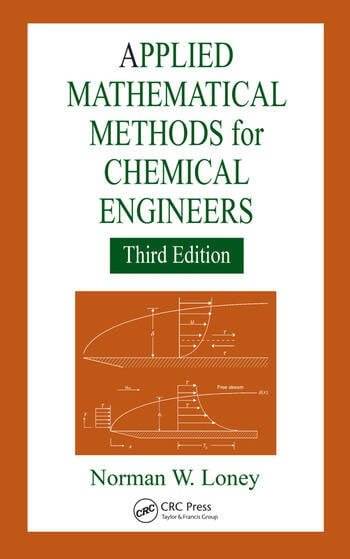# Applied Mathematical Methods for Chemical Engineers

## 3rd Edition

Norman W. Loney

CRC Press
Published October 5, 2015
Textbook - 545 Pages - 47 B/W Illustrations
ISBN 9781466552999 - CAT# K15249

For Instructors Request Inspection Copy

USD\$145.00

FREE Standard Shipping!

## Preview

### Summary

Focusing on the application of mathematics to chemical engineering, Applied Mathematical Methods for Chemical Engineers addresses the setup and verification of mathematical models using experimental or other independently derived data. The book provides an introduction to differential equations common to chemical engineering, followed by examples of first-order and linear second-order ordinary differential equations. Later chapters examine Sturm–Liouville problems, Fourier series, integrals, linear partial differential equations, regular perturbation, combination of variables, and numerical methods emphasizing the method of lines with MATLAB® programming examples.

Fully revised and updated, this Third Edition:

• Includes additional examples related to process control, Bessel Functions, and contemporary areas such as drug delivery
• Introduces examples of variable coefficient Sturm–Liouville problems both in the regular and singular types
• Demonstrates the use of Euler and modified Euler methods alongside the Runge–Kutta order-four method
• Inserts more depth on specific applications such as nonhomogeneous cases of separation of variables
• Adds a section on special types of matrices such as upper- and lower-triangular matrices
• Presents a justification for Fourier-Bessel series in preference to a complicated proof
• Incorporates examples related to biomedical engineering applications
• Illustrates the use of the predictor-corrector method
• Expands the problem sets of numerous chapters

Applied Mathematical Methods for Chemical Engineers, Third Edition uses worked examples to expose several mathematical methods that are essential to solving real-world process engineering problems.

#### Instructors

We provide complimentary e-inspection copies of primary textbooks to instructors considering our books for course adoption.